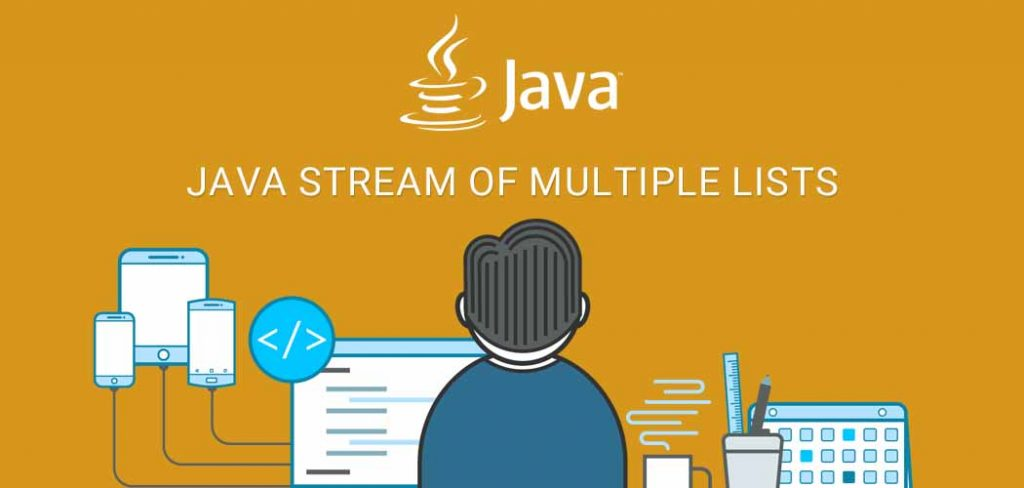# Java Stream of Multiple Lists

## Overview

In Java 8, we know how to get a stream of List using stream() or parrallelStream() method of Collection interface. But what if we want a stream of two List or stream of List of Lists as a single logical stream to combine/merge those lists as one or do the same process on all. So, this article is going to show you how to get Java Stream of Multiple Lists using Java 8 Stream API (Stream interface) with an example.

## Example 1: Using the Stream.concat() Method

Stream.concat() is a static method, it combines two given streams into one logical stream of all elements of the first list followed by all elements of the second list.

Let’s see example for how to combine two integer type list stream into new one integer type stream list using the concat() method.

```List<Integer> odd = Arrays.asList(1, 3, 5, 7, 9);
List<Integer> even = Arrays.asList(2, 4, 6, 8, 10);
Stream<Integer> resultStream = Stream.concat(odd.stream(), even.stream()); //Concatenate stream of odd with stream of even
resultStream.forEach(System.out::println);```

### Output

```1     //odd list elements
3
5
7
9
2     //even list elements
4
6
8
10```

If you want to combine/merge more than two list, need to call concat() method one time less than a number of lists.

See the following example for how to combine a stream of three list using two times concat() method.

```List<Integer> odd = Arrays.asList(1, 3, 5, 7, 9);
List<Integer> even = Arrays.asList(2, 4, 6, 8, 10);
List<Integer> armstrongNo = Arrays.asList(0, 1, 153, 370, 371);
Stream<Integer> firstStream = Stream.concat(odd.stream(), even.stream());  //Merge stream of odd and even list
Stream<Integer> finalStream = Stream.concat(firstStream, armstrongNo.stream());  //Merge fisrtStream and stream of armstrongNo
finalStream.forEach(System.out::println);```

### Output

```1     //odd list elements
3
5
7
9
2     //even list elements
4
6
8
10
0    //armstrongNo list elements
1
153
370
371```

## Example 2: Using the Stream.flatMap() Method

The flatMap() applies the given mapping function to each element of collection stream and returns a new Stream of result elements of applied given mapping function.

See the following example for how to merge two collections using the flatMap() method.

```List<Integer> odd = Arrays.asList(1, 3, 5, 7, 9);
List<Integer> even = Arrays.asList(2, 4, 6, 8, 10);
Stream<Integer> resultStream = Stream.of(odd, even)    // Stream.of() accept one or more list as a argument
.flatMap(Collection::stream);                  //Or .flatMap(list -> list.stream())
//Here, resultStream contains all elements of odd followed by elements of even
resultStream.forEach(System.out::println);```

### Output

Here, the output is the same as the previous example, both implementation behaves the same.

```1     //odd list elements
3
5
7
9
2     //even list elements
4
6
8
10```

See following example for how to combine List of List using the flatMap() method.

```List<Integer> odd = Arrays.asList(1, 3, 5, 7, 9);
List<Integer> even = Arrays.asList(2, 4, 6, 8, 10);
List<Integer> armstrongNo = Arrays.asList(0, 1, 153, 370, 371);
List<List<Integer>> listOfList = new ArrayList<>();
Stream<Integer> integerStream = listOfList.stream()
.flatMap(Collection::stream);         //Here, All current stream elements mapped to stream
//OR: .flatMap(list -> list.stream())
integerStream.forEach(System.out::println);   //Print all elements of sub lists: odd, even and armstrongNo```

### Output

```1     //odd list elements
3
5
7
9
2     //even list elements
4
6
8
10
0    //multiplesOfTen list elements
1
153
370
371```

## Conclusion

We have seen, how to get Java Stream of Multiple Lists using Java 8 Stream API (Stream interface) with an example. Same way we can do it with other Collection implementations.